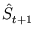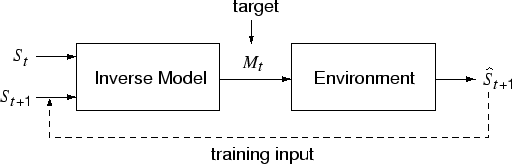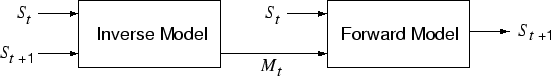Next: 1.5.4 Recurrent neural networks Up: 1.5 Learning of sensorimotor Previous: 1.5.2 Internal models

## 1.5.3 Feed-forward networks as internal models

This section describes internal models that are built with multi-layer perceptrons. Forward models can be learned by observing the effectof actions Mt on the environment. This effectis the target value in the training (Jordan and Rumelhart, 1992).

For inverse models, actions and effects exchange their role as input and output. One approach to learn an inverse model is `direct inverse modeling' (Jordan and Rumelhart, 1992). Here, the environment produces input patterns instead of target values (figure 1.3). The action Mt will result in the sensory state. Thus, the inverse model can be trained to maponto Mt. Training data can be produced by randomly sampling the action space (Kuperstein, 1988).However, this approach will fail if the environment maps different motor commands onto the same sensory state. The inverse model cannot learn the corresponding one-to-many mapping because the MLP would average across the many possible motor commands, and such an average might not be a desirable solution (Movellan and McClelland, 1993). Therefore, Jordan and Rumelhart (1992) suggested to link the inverse model with a forward model (figure 1.4). First, the forward model is trained separately, as described above. Then, the combined network learns an identity mapping (note, in figure 1.4, St+1 acts both as an input and as a target). In the learning process, the weights of the inverse model are adjusted using error backpropagation, while the error propagates through the forward model without changing its weights.Next: 1.5.4 Recurrent neural networks Up: 1.5 Learning of sensorimotor Previous: 1.5.2 Internal models
Heiko Hoffmann
2005-03-22